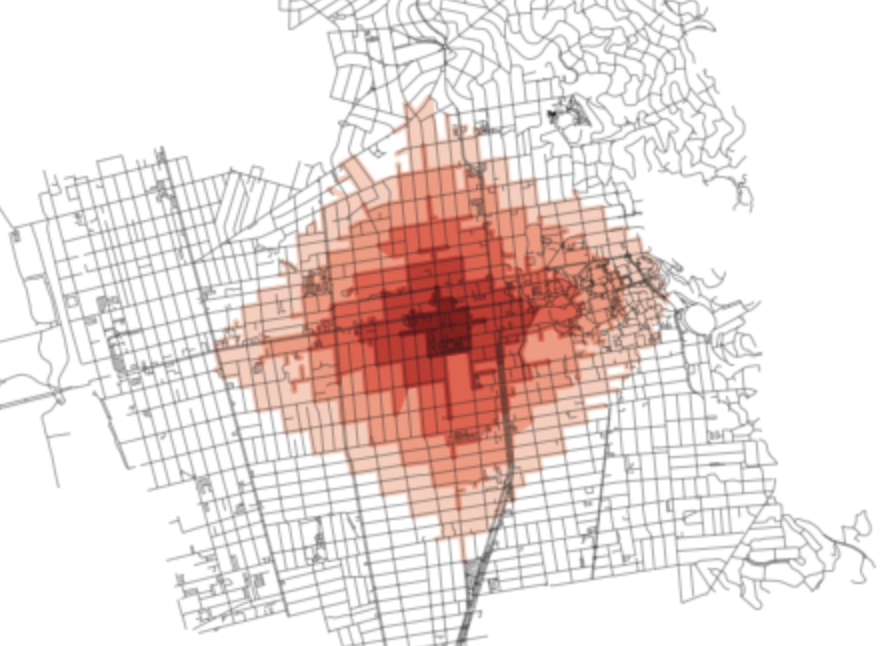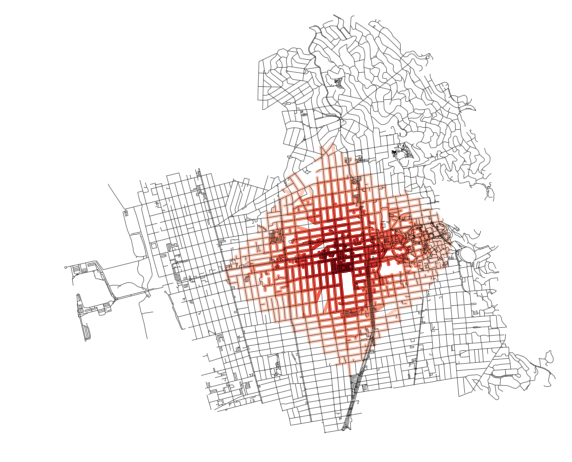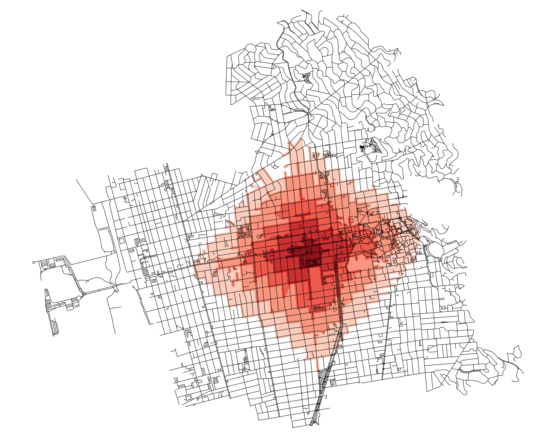/ osmnx-examples Public

# Optional method of better rendering ischrones for accessibility using nodes + edges#4

Closed
opened this issue Dec 16, 2017 · 6 comments
Closed

# Optional method of better rendering ischrones for accessibility using nodes + edges #4

opened this issue Dec 16, 2017 · 6 comments

### kuanb commented Dec 16, 2017

 Regarding this example: https://github.com/gboeing/osmnx-examples/blob/master/notebooks/13-isolines-isochrones.ipynb I propose a new method, `make_iso_polys`, outlined below. This, rather than a convex hull, might best demonstrate coverage area while preserved nuances ("inlets" or areas of inaccessibility not captured when creating a convex hull around a point cloud). Example of what improved plot looks like:Ful function defintion. ``````def make_iso_polys(G, edge_buff=25, node_buff=50): isochrone_polys = [] for trip_time in sorted(trip_times, reverse=True): subgraph = nx.ego_graph(G, center_node, radius=trip_time, distance='time') node_points = [Point((data['x'], data['y'])) for node, data in subgraph.nodes(data=True)] nodes_gdf = gpd.GeoDataFrame({'id': subgraph.nodes()}, geometry=node_points) nodes_gdf = nodes_gdf.set_index('id') edge_lines = [] for n_fr, n_to in subgraph.edges(): f = nodes_gdf.loc[n_fr].geometry t = nodes_gdf.loc[n_to].geometry edge_lines.append(LineString([f,t])) n = nodes_gdf.buffer(node_buff).geometry e = gpd.GeoSeries(edge_lines).buffer(edge_buff).geometry all_gs = list(n) + list(e) new_iso = gpd.GeoSeries(all_gs).unary_union isochrone_polys.append(new_iso) return isochrone_polys `````` Further elaboration available here: http://kuanbutts.com/2017/12/16/osmnx-isochrones/ The text was updated successfully, but these errors were encountered:changed the title Optional method of rendering ischrones Optional method of better rendering ischrones for accessibility using nodes + edges Dec 16, 2017

### gboeing commented Dec 18, 2017

 @kuanb nice. How did you parameterize `edge_buff` and `node_buff` for the example image?

### kuanb commented Dec 18, 2017

 Those are both buffered at 25 (meters). In the final example at the bottom of http://kuanbutts.com/2017/12/16/osmnx-isochrones/ I use 25 on edges and 50 on nodes (the above defaults).

### gboeing commented Dec 18, 2017 • edited

 I like how the blocks are filled-in in your example image above. But, I'm not seeing the same results when I parameterize your sample code that way: ```def make_iso_polys(G, edge_buff=25, node_buff=50): isochrone_polys = [] for trip_time in sorted(trip_times, reverse=True): subgraph = nx.ego_graph(G, center_node, radius=trip_time, distance='time') node_points = [Point((data['x'], data['y'])) for node, data in subgraph.nodes(data=True)] nodes_gdf = gpd.GeoDataFrame({'id': subgraph.nodes()}, geometry=node_points) nodes_gdf = nodes_gdf.set_index('id') edge_lines = [] for n_fr, n_to in subgraph.edges(): f = nodes_gdf.loc[n_fr].geometry t = nodes_gdf.loc[n_to].geometry edge_lines.append(LineString([f,t])) n = nodes_gdf.buffer(node_buff).geometry e = gpd.GeoSeries(edge_lines).buffer(edge_buff).geometry all_gs = list(n) + list(e) new_iso = gpd.GeoSeries(all_gs).unary_union isochrone_polys.append(new_iso) return isochrone_polys isochrone_polys = make_iso_polys(G, edge_buff=25, node_buff=25) fig, ax = ox.plot_graph(G, fig_height=8, show=False, close=False, edge_color='k', edge_alpha=0.2, node_color='none') for polygon, fc in zip(isochrone_polys, iso_colors): patch = PolygonPatch(polygon, fc=fc, ec='none', alpha=0.6, zorder=-1) ax.add_patch(patch) plt.show()```Does it make sense for 25-meter buffers to fill in the blocks, as seen in your image? They are much larger than 25x25 meters, so this output I'm seeing makes sense with those parameters.

### kuanb commented Dec 20, 2017

 So sorry and thanks for pointing this out. I updated the post and also am including the fixed script here. I forgot to add on step to the method. I acknowledge this won't always work if you have a "donut" situation. Would need to think about a better way to handle such situations... ``````def make_iso_polys(G, edge_buff=25, node_buff=50, infill=False): isochrone_polys = [] for trip_time in sorted(trip_times, reverse=True): subgraph = nx.ego_graph(G, center_node, radius=trip_time, distance='time') node_points = [Point((data['x'], data['y'])) for node, data in subgraph.nodes(data=True)] nodes_gdf = gpd.GeoDataFrame({'id': subgraph.nodes()}, geometry=node_points) nodes_gdf = nodes_gdf.set_index('id') edge_lines = [] for n_fr, n_to in subgraph.edges(): f = nodes_gdf.loc[n_fr].geometry t = nodes_gdf.loc[n_to].geometry edge_lines.append(LineString([f,t])) n = nodes_gdf.buffer(node_buff).geometry e = gpd.GeoSeries(edge_lines).buffer(edge_buff).geometry all_gs = list(n) + list(e) new_iso = gpd.GeoSeries(all_gs).unary_union # If desired, try and "fill in" surrounded # areas so that shapes will appear solid and blocks # won't have white space inside of them if infill: new_iso = Polygon(new_iso.exterior) isochrone_polys.append(new_iso) return isochrone_polys ``````

### kuanb commented Dec 20, 2017 • edited

 Here's the result of the following script: ``````isochrone_polys = make_iso_polys(G, 25, 0, True) fig, ax = ox.plot_graph(G, fig_height=8, show=False, close=False, edge_color='k', edge_alpha=0.2, node_color='none') for polygon, fc in zip(isochrone_polys, iso_colors): patch = PolygonPatch(polygon, fc=fc, ec='none', alpha=0.6, zorder=-1) ax.add_patch(patch) plt.show() ``````### gboeing commented Dec 21, 2017

 thanks @kuanb I've added a demo of this to the notebook!